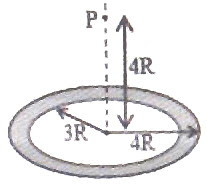## Wednesday, April 14, 2010

### IIT-JEE 2010 Questions on Gravitation

I would never die for my belief because I might be wrong.

– Bertrand Russell

Two questions on gravitation were included in the IIT-JEE 2010 question paper. They are given below with solution. The first question is a ‘single correct choice type’ (single answer type multiple choice) question where as the second question is an ‘integer type’ question which has a single digit integer ranging from 0 to 9 as answer.(1) A thin uniform annular disc (see figure) of mass M has outer radius 4R and inner radius 3R. The work required to take a unit mass from point P on its axis to infinity is

(A) (2GM /7R) (42 5)

(B) (2GM /7R) (42 5)

(C) GM /4R

(D) (2GM /5R) (2 – 1)

The work required to take a unit mass from infinity to point P is the gravitational potential at P and it will be negative. Therefore, the work required to take a unit mass from point P to infinity will be numerically equal to the gravitational potential at P but it will be positive.

The annular disc can be considered to be made of a large number of concentric rings of radii ranging from 3R to 4R. Consider one such ring of radius r and small thickness dr. Its mass dm is given by

dm = [M/π(16R2 – 9R2)] 2πr dr = 2Mrdr/7R2

If the rim of the ring is at distance x from the point P, the gravitational potential (dV) at P due to the ring is given by

dV = Gdm/x = – (2GM/7R2)(rdr/x)

But x = (16R2 + r2)1/2 and so we have dx= (½ )×(16R2 + r2)1/2 ×2rdr

Or, dx = rdr/(16R2 + r2)1/2 = rdr/x

Therefore dV = – (2GM/7R2)dx

The gravitational potential at P due to the entire annular disc is given by

V = dV = (2GM/7R2)dx = – (2GM/7R2)[x]

The integration is between the appropriate limits of x, which are (16R2 + 9R2)1/2 and (16R2 + 16R2)1/2, on substituting the values r = 3R and r = 4R respectively in the expression, x = (16R2 + r2)1/2. Therefore we have

V = – (2GM/7R2)[(16R2 + 16R2)1/2 (16R2 + 9R2)1/2]

= – (2GM/7R2)[R√(32) R√(25)]

Thus V = (2GM/7R) (42 5)

The negative sign is to be dispensed with to obtain the work required to take a unit mass from point P to infinity and the correct choice is (2GM/7R) (42 5).

(2) Gravitational acceleration on the surface of a planet is (√6/11)g, where g is the gravitational acceleration on the surface of the earth. The average mass density of the planet is 2/3 times that of the earth. If the escape speed on the surface of the earth is taken to be 11 kms–1, the escape speed on the surface of the planet in kms–1 will be :

[This is is an ‘integer type’ question. You have to work out this question and the single digit integer answer is to be shown in the Objective Response Sheet (ORS) by darkening the appropriate bubble].

We have vesc = √(2gR) where g is the acceleration due to gravity and R is the radius of the planet (or earth or star).

Therefore vesc α √(gR).

If gp and ge are the accelerations due to gravity on the surface of the planet and the earth, Rp and Re are their radii, and gp and ge the escape velocities respectively, we have

vp/ve = √( gpRp)/ √( geRe) = √[( gp/ge)(Rp/Re)] ………(i)

Since the gravitational acceleration on the surface is given by g = GM/R2 where G is the gravitational constant and M is the mass of the planet (or earth or star), we can write

g = G[(4/3)πR3ρ] /R2 where ρ is the average mass density of the planet (or earth or star).

Therefore g α and gp/ge = (Rp/Re)(ρp/ρe).

From this Rp/Re = (gp/ge)/(ρp/ρe).

But gp/ge = √6/11 and ρp/ρe = 2/3 as given in the question. Therefore we have

Rp/Re = (√6/11)×3/2

Substituting these in Eq(i),

vp/ve = √[(√6/11) (√6/11)×3/2] = (√6/11) ×√(3/2) = 3/11

Since ve = 11 kms–1, the escape speed on the surface of the planet in kms–1 will be

vp = 11×(3/11) = 3 kms–1

1.Anonymous10:46 AM

i am satisfied wid ur answer

2.Thanks

3.Anonymous10:25 AM

It helped. Thanks :-)

1.Thanks